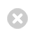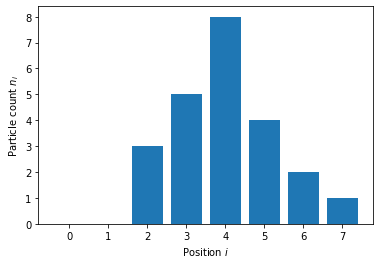Close

MPHY0021: Research Software Engineering With Python

Home## Classroom exercise: energy calculation¶

### Diffusion model in 1D¶

Description: A one-dimensional diffusion model. (Could be a gas of particles, or a bunch of crowded people in a corridor, or animals in a valley habitat...)

• Agents are on a 1d axis
• Agents do not want to be where there are other agents
• This is represented as an 'energy': the higher the energy, the more unhappy the agents.

Implementation:

• Given a vector $n$ of positive integers, and of arbitrary length
• Compute the energy, $E(n) = \sum_i n_i(n_i - 1)$
• Later, we will have the likelyhood of an agent moving depend on the change in energy.
In :
import numpy as np
from matplotlib import pyplot as plt
%matplotlib inline

density =  np.array([0, 0, 3, 5, 8, 4, 2, 1])
fig, ax = plt.subplots()
ax.bar(np.arange(len(density)), density)
ax.xrange=[-0.5, len(density)-0.5]
ax.set_ylabel("Particle count $n_i$")
ax.set_xlabel("Position $i$")

Out:
Text(0.5, 0, 'Position $i$')Here, the total energy due to position 2 is $3 (3-1)=6$, and due to column 7 is $1 (1-1)=0$. We need to sum these to get the total energy.

### Starting point¶

Create a Python module:

In :
%%bash
mkdir -p diffusion
touch diffusion/__init__.py

• Implementation file: diffusion_model.py
In :
%%writefile diffusion/model.py
def energy(density, coeff=1.0):
"""
Energy associated with the diffusion model

Parameters
----------

density: array of positive integers
Number of particles at each position i in the array
coeff: float
Diffusion coefficient.
"""
# implementation goes here

Writing diffusion/model.py

• Testing file: test_diffusion_model.py
In :
%%writefile diffusion/test_model.py
from .model import energy
def test_energy():
""" Optional description for reporting """
# Test something

Writing diffusion/test_model.py


Invoke the tests:

In :
%%bash
cd diffusion
pytest

============================= test session starts ==============================
platform linux -- Python 3.8.12, pytest-6.2.5, py-1.11.0, pluggy-1.0.0
rootdir: /home/runner/work/rsd-engineeringcourse/rsd-engineeringcourse/ch03tests/diffusion
plugins: cov-3.0.0
collected 1 item

test_model.py .                                                          [100%]

============================== 1 passed in 0.02s ===============================


Now, write your code (in model.py), and tests (in test_model.py), testing as you do.

### Solution¶

Don't look until after you've tried!

In :
%%writefile diffusion/model.py
"""  Simplistic 1-dimensional diffusion model """

def energy(density):
"""
Energy associated with the diffusion model

:Parameters:

density: array of positive integers
Number of particles at each position i in the array/geometry
"""
from numpy import array, any, sum

# Make sure input is an numpy array
density = array(density)

# ...of the right kind (integer). Unless it is zero length,
#    in which case type does not matter.

if density.dtype.kind != 'i' and len(density) > 0:
raise TypeError("Density should be a array of *integers*.")
# and the right values (positive or null)
if any(density < 0):
raise ValueError("Density should be an array of *positive* integers.")
if density.ndim != 1:
raise ValueError("Density should be an a *1-dimensional*" +
"array of positive integers.")

return sum(density * (density - 1))

Overwriting diffusion/model.py

In :
%%writefile diffusion/test_model.py
""" Unit tests for a diffusion model """

from pytest import raises
from .model import energy

def test_energy_fails_on_non_integer_density():
with raises(TypeError) as exception:
energy([1.0, 2, 3])

def test_energy_fails_on_negative_density():
with raises(ValueError) as exception: energy(
[-1, 2, 3])

def test_energy_fails_ndimensional_density():
with raises(ValueError) as exception: energy(
[[1, 2, 3], [3, 4, 5]])

def test_zero_energy_cases():
# Zero energy at zero density
densities = [ [], , [0, 0, 0] ]
for density in densities:
assert energy(density) == 0

def test_derivative():
from numpy.random import randint

# Loop over vectors of different sizes (but not empty)
for vector_size in randint(1, 1000, size=30):

# Create random density of size N
density = randint(50, size=vector_size)

# will do derivative at this index
element_index = randint(vector_size)

# modified densities
density_plus_one = density.copy()
density_plus_one[element_index] += 1

# Compute and check result
# d(n^2-1)/dn = 2n
expected = (2.0 * density[element_index]
if density[element_index] > 0
else 0 )
actual = energy(density_plus_one) - energy(density)
assert expected == actual

def test_derivative_no_self_energy():
""" If particle is alone, then its participation to energy is zero """
from numpy import array

density = array([1, 0, 1, 10, 15, 0])
density_plus_one = density.copy()
density += 1

expected = 0
actual = energy(density_plus_one) - energy(density)
assert expected == actual

Overwriting diffusion/test_model.py

In :
%%bash
cd diffusion
pytest

============================= test session starts ==============================
platform linux -- Python 3.8.12, pytest-6.2.5, py-1.11.0, pluggy-1.0.0
rootdir: /home/runner/work/rsd-engineeringcourse/rsd-engineeringcourse/ch03tests/diffusion
plugins: cov-3.0.0
collected 6 items

test_model.py ......                                                     [100%]

============================== 6 passed in 0.12s ===============================


### Coverage¶

With py.test, you can use the "pytest-cov" plugin to measure test coverage

In :
%%bash
cd diffusion
pytest --cov="diffusion"

============================= test session starts ==============================
platform linux -- Python 3.8.12, pytest-6.2.5, py-1.11.0, pluggy-1.0.0
rootdir: /home/runner/work/rsd-engineeringcourse/rsd-engineeringcourse/ch03tests/diffusion
plugins: cov-3.0.0
collected 6 items

test_model.py ......                                                     [100%]

---------- coverage: platform linux, python 3.8.12-final-0 -----------
Name            Stmts   Miss  Cover
-----------------------------------
__init__.py         0      0   100%
model.py           10      0   100%
test_model.py      31      0   100%
-----------------------------------
TOTAL              41      0   100%

============================== 6 passed in 0.19s ===============================


Or an html report:

In :
%%bash
cd diffusion
pytest --cov="diffusion" --cov-report html

============================= test session starts ==============================
platform linux -- Python 3.8.12, pytest-6.2.5, py-1.11.0, pluggy-1.0.0
rootdir: /home/runner/work/rsd-engineeringcourse/rsd-engineeringcourse/ch03tests/diffusion
plugins: cov-3.0.0
collected 6 items

test_model.py ......                                                     [100%]

---------- coverage: platform linux, python 3.8.12-final-0 -----------
Coverage HTML written to dir htmlcov

============================== 6 passed in 0.20s ===============================


Look at the coverage results

In [ ]: